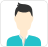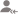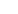true

Take Class 12 Tuition from the Best Tutors

•Affordable fees
•1-1 or Group class
•Flexible Timings
•Verified Tutors

Search inLearn Class XI-XII Tuition (PUC) with Free Lessons & Tips

Post a Lesson

All

All

Lessons

Discussion

Lesson Posted on 18/10/2021 Learn Business StudiesPramod

y' = Lt / x x => 0 Therefore if y = sin x y' = Lt / x x=>0 y' = Lt / x x => 0 =>Lt / x x => 0 => Lt / x x => 0s =>sin x Lt(cos x-1)/ x+cos xLt(sin x/ x) x => 0 x=> 0 => sin... read more

y' = Lt   [f(x+∆x) - f(x)]/∆x

∆x => 0

Therefore if y = sin x

y' = Lt  [sin (x + ∆x) - sin x] / ∆x

∆x=>0

y' = Lt [sin x cos ∆x+cos x sin ∆x - sin x]/∆x

∆x => 0

=>Lt [ sin x cos ∆x - sin x + cos x sin ∆x]/∆x

∆x => 0

=> Lt [ sin x (cos ∆x - 1) + cos x sin ∆x]/ ∆x

∆x => 0s

=>sin x Lt(cos ∆x-1)/∆x+cos xLt(sin ∆x/∆x)

∆x => 0                         ∆x=> 0

=> sin x • 0 + cos x• 1

=> dy/dx (sin x) = 0 + cos x

=> dy/dx (sin x ) = cos x

[ Lt sin x/x = 1 ] & Lt (cos x - 1)/x = 1-1 = 0]

x => 0                      x => 0

Dislike Bookmark

Lesson Posted on 09/04/2020 Learn BBA Tuition +4 BCom Tuition Accountancy Accounts Unit 1-Financial Accounting(Part A)Theoretical FrameworkWith over more than ten years of experience as a tutor for accountancy, finance, Taxation, Costing and...

Dear Students, Final Accounts question is a very scoring question in the accountancy Paper and is also a compulsory question for most of the boards. So here is the list of simple steps to be follwed while solving a final Accounts sum: 1. Read the list of Trial Balance items and adjustments carefully 2.... read more

Dear Students,

Final Accounts question is a very scoring question in the accountancy Paper and is also a compulsory question for most of the boards.

So here is the list of simple steps  to be follwed while solving a final Accounts sum:

2. Record all the debit items given in the Trial Balance on either expenses side of Trading-P&L Account or Asset Side of Balancesheet

3. Record all the credit items given in the Trial Balance on either Income side of Trading-P&L Account or Liabilities Side of Balancesheet

4. Remember to post all the trial balance items only once, but all the adjustments items twice (one debit effect and one credit effect)

6. Add this profit obtained with the Capital, on the liabilities side of the balance sheet

7. Take the total of the balance sheet.

Remember every debit has a corresponding credit.

Dislike Bookmark

Answered on 23/07/2021 Learn Class XI-XII Tuition (PUC) +1Joy Mukherjee

To find the average speed, you need to know the formula of average speed. The avg speed 0= 2 d / (t1+ t2) = 2 v1.v2/(V1 + V2). Just substitute the value.
Dislike Bookmark

Take Class 12 Tuition from the Best Tutors

•Affordable fees
•Flexible Timings
•Choose between 1-1 and Group class
•Verified Tutors

Lesson Posted on 06/06/2017 Learn Class XI-XII Tuition (PUC)Mahesh

I am a well experienced, qualified teacher and tutor teaching CBSE Accounts having 17 years of experience...

Interest on partners loan: It is a charge against profits. It is provided irrespective of profits or loss. It will also be provided in the absence of Partnership Deed @ 6% per annum. The following entries are passed to record the interest on partner’s loan: For allowing Interest on loan: Interest... read more

Interest on partners loan: It is a charge against profits. It is provided irrespective of profits or loss. It will also be provided in the absence of Partnership Deed @ 6% per annum. The following entries are passed to record the interest on partner’s loan:

• For allowing Interest on loan: Interest on Partner’s Loan A/c Dr. To Partner’s Loan A/c (Being interest on loan allowed @___% p.a.)
• For transferring Interest on Loan to Profit and Loss A/c: Profit and Loss A/c Dr. To Interest on Loan A/c (Being interest on loan transferred to P & L A/c) It is always DEBITED to Profit and Loss A/c Rent paid to a partner is also a charge against profits and it will also be DEBITED to Profit and Loss A/c.

Example: A and B entered into partnership on 1st April, 2010 without any partnership deed. They
introduced capitals of Rs. 5, 00,000 and Rs. 3, 00,000 respectively. On 31st October,
2010, A advanced Rs. 2, 00,000 by way of loan to the firm without any agreement as to
interest. The Profit and Loss Account for the year ended 31032011
showed a profit of Rs. 4,30,000 but the partners could not agree upon the amount of interest on Loan to be
charged and the basis of division of profits. Pass a Journal Entry for the distribution of the Profits between the partners and prepare the Capital A/cs of both the partners and Loan A/c of ‘A’.

Solution: Profit and Loss Appropriation Account. For the year ending on 31st March, 2011 Dr. Cr. Particulars "Particulars". To Profits transferred to By Profit and Loss A/c Capital A/c of A : Rs. 2,12,500 (Net Profits 4,30,000) B: Rs 2,12,500. Less : Interest on Rs 4,25,000 A's Loan (5,000) Rs 4,25,000 Rs 4,25,000 Rs 4,25,000 Dr. Partner's Capital A/cs Cr Date Particular A B Date Particular A B 31.03.2011 To 01.04.2010 By Bank A/c 5,00,000 3,00,000 Balance 7,12,500 5,12,500 31.03.2011 By Profit and c/d Loss Appropriation A/c 2,12,500 2,12,500 7,12,500 5,12,500 7,12,500 5,12,500. JOURNAL Date Particulars L.F. Debit Credit Rs. Rs. 31.03.2011 Profit and Loss Appropriation A/c Dr 4,25,000 To A's Capital A/c 2,12,500To B's Capital A/c 2,12,500 (Being profit distributed among the partners) Dr. A's Loan A/c Cr.
Date Particulars Amount Date Particulars Amount Rs. 2011 To Balance c/d 2,05,000 2010 March, Oct., 31 By Bank A/c 2,00,000 31 2011 March, 31 By Interest on Loan A/c 5,000 2,05,000 2,05,000. Note : Interest on A's Loan = Loan Amount x Rate /100x Time Left after Loan Taken/12 = 2,00,000x 6/100x 05/12 = Rs.5,000.

Dislike Bookmark

Answered on 12/06/2021 Learn Class XI-XII Tuition (PUC)Rajeev

89
Dislike Bookmark

Answered on 27/02/2021 Learn Class XI-XII Tuition (PUC)Rajan Mittal

CBSE JEE NEET B.TECH Teacher with an experience of 20+ years

These are a form of electromagnetic waves of very short wavelength. Based on radiation's dual nature, they may be treated as a stream of high energy particles called photons. Photons are electrically neutral.
Dislike Bookmark

Take Class 12 Tuition from the Best Tutors

•Affordable fees
•Flexible Timings
•Choose between 1-1 and Group class
•Verified Tutors

Answered on 31/12/2019 Learn Class XI-XII Tuition (PUC)Vishwajeet

Increases
Dislike Bookmark

Answered on 04/07/2015 Learn Class XI-XII Tuition (PUC)Jitendra Suhalka

Software engineer

Given data: Current I1 and I2 = 100 Amps; Distance = 20 mm = 20 10-3 m; Force = ? We know that the force is found by the following expression. Torque developed force fT=M0 *I1 *I2/(2*(Pi)*d)=4*(Pi)*(10)^-7 *(100)^2/2*(pi)*20*(10)^-3=0.1 N
Dislike Bookmark

Answered on 13/06/2021 Learn Class XI-XII Tuition (PUC)Nidhin

i= sin wt f= 25 HZ w= 2×pi×f t=? 50=100 sin wt 50=100 sin (2×180×25) t 50/100= sin 9000 t 1/2=sin 9000 t Sin-' (1/2)= 9000t sin -' (1/2)=30 30= 9000 t t = 30/9000 t = 1/300 = 3.33 ms. read more

i= sin wt

f= 25 HZ

w= 2×pi×f

t=?

50=100 sin wt

50=100 sin (2×180×25) t

50/100= sin 9000 t

1/2=sin 9000 t

Sin-' (1/2)= 9000t                             sin -' (1/2)=30

30= 9000 t

t = 30/9000

t = 1/300

= 3.33 ms.

Dislike Bookmark

Take Class 12 Tuition from the Best Tutors

•Affordable fees
•Flexible Timings
•Choose between 1-1 and Group class
•Verified Tutors

Answered on 20/03/2020 Learn Class XI-XII Tuition (PUC)Charlie

Nodes and antinodes are known to form stationary waves. In a given stationary wave, the distance between any given two successive nodes is half the wavelength. The approximate distance between a node and the immediate next antinode is actually one-fourth of a given wavelength. In other words, the total... read more

Nodes and antinodes are known to form stationary waves. In a given stationary wave, the distance between any given two successive nodes is half the wavelength. The approximate distance between a node and the immediate next antinode is actually one-fourth of a given wavelength.

In other words, the total distance or gap between two consecutive node and an antinode in a given current wave is usually represented as the half the length of the wave of the entire waves produced.

The formula is Distance= lambda/2

Where lambda is the wavelength. It is also to be known that the standing wave varies in distance proportionately to its wavelength with the displacement being zero always

I hope this helps.

Dislike Bookmark

UrbanPro.com helps you to connect with the best Class 12 Tuition in India. Post Your Requirement today and get connected.

Overview

Questions 4.2 k

Lessons 468

Total Shares664,836 Followers

Class 12 Tuition in:

x

X

Looking for Class 12 Tuition Classes?

The best tutors for Class 12 Tuition Classes are on UrbanPro

• Select the best Tutor
• Book & Attend a Free Demo
• Pay and start LearningTake Class 12 Tuition with the Best Tutors

The best Tutors for Class 12 Tuition Classes are on UrbanProUrbanPro.com is India's largest network of most trusted tutors and institutes. Over 55 lakh students rely on UrbanPro.com, to fulfill their learning requirements across 1,000+ categories. Using UrbanPro.com, parents, and students can compare multiple Tutors and Institutes and choose the one that best suits their requirements. More than 7.5 lakh verified Tutors and Institutes are helping millions of students every day and growing their tutoring business on UrbanPro.com. Whether you are looking for a tutor to learn mathematics, a German language trainer to brush up your German language skills or an institute to upgrade your IT skills, we have got the best selection of Tutors and Training Institutes for you. Read more